### LESSON PLANS AND RESOURCES FOR TEACHING MULTIPLY TENS, HUNDREDS, AND THOUSANDS!

Teaching How to Multiply Tens, Hundreds, and Thousands can be a lot of FUN!

Since 4th graders know how to multiply by 1 and multiply by 10, today's lesson is mostly a tip and a shortcut since your students already understand the "why" of the math skill.

The Essential Question is How Does Understanding Place Value Help You Multiply Tens, Hundreds, and Thousands?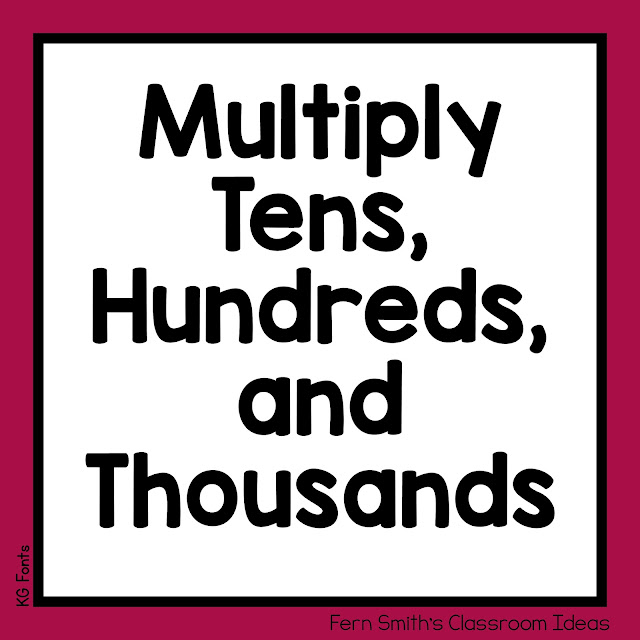By now your students have studied the shortcuts for multiplying any number by 10, 100, or 1,000, but still understand the "why?" and the "how?" it all works. Today's lesson is about

shortcuts.

Introduction to How Multiply Tens, Hundreds, and Thousands

1. Review a few basic multiplication problems, on the board, or on your students' white boards.

3 x 1 = 3

3 x 10 = 30

3 x 100 = 300

12 x 1 = 12

12 x 10 = 120

12 x 100 = 1,200

To multiply any number by 10, just add ONE zero on the end.

To multiply any number by 100, just add TWO zeros on the end.

To multiply any number by 1,000, just add THREE zeros on the end.

The important part of this tip is to make sure your students are placing the exact number of zeros that are needed.

To review and check for understanding, use this example.

10 x 1 = 10

10 x 10 = 100

10 x 100 = 1,000

Discuss that the MAIN number you are multiplying by is 10, which already has a zero, then add on the EXTRA number of zeros from there.

2. Moving to other numbers, for example, 2, 20, 200...

What is 20 × 8?

Imagine the problem without the zero. Then it just becomes 2 × 8 = 16. Then, just add a zero to the 16, for 20 x 8=  so now the answer is 160. 200 x 8 = 1,600.

3. Why does the shortcut work?

It works because you can multiply in any order.

When multiplying by 40, we can change the 40 into 4 x 10 =.

Example:

40 × 11 = 10 × 4 × 11

First multiply 4 × 11 = 44. Then, multiply by 10 × 44, getting 440 as your answer.

Practice Problems For Multiplying Tens, Hundreds, and Thousands

1. 4 x 3 =

40 x 3 =

400 x 3 =

2. 5 x 5 =

50 x 5 =

500 x 5 =

3. 8 x 4 =

80 x 4 =

800 x 4 =

4. 9 x 7 =

90 x 7 =

900 x 7 =

5. 2 x 2 =

20 x 2 =

200 x 2 =

Answers For the Practice Problems For Multiplying Tens, Hundreds, and Thousands

1. 4 x 3 = 12

40 x 3 = 120

400 x 3 = 1,200

2. 5 x 5 = 25

50 x 5 = 250

500 x 5 = 2,500

3. 8 x 4 = 32

80 x 4 = 320

800 x 4 = 3,200

4. 9 x 7 = 63

90 x 7 = 630

900 x 7 = 6,300

5. 2 x 2 = 4

20 x 2 = 40

200 x 2 = 400

BONUS

Write a number sentence for each question.

1. One hour has ______ minutes.

How many minutes are in 12 hours?

How many minutes are in 24 hours?

2. One hour has ______ minutes, and one minute has ______ seconds.

How many seconds are there in one hour?

Write a number sentence for each question.

1. One hour has 60 minutes. 1 x 60 = 60

How many minutes are in 12 hours? 12 x 60 = 720

How many minutes are in 24 hours? 24 x 60 = 1,440

2. One hour has 60 minutes, and one minute has 60 seconds.

How many seconds are there in one hour? 60 x 60 = 3,600

MORE RESOURCES TO HELP TEACH AND REVIEW MULTIPLY TENS, HUNDREDS, AND THOUSANDSFourth Grade Math Multiply Tens, Hundreds, and Thousands Center Games and Task Cards

This Fourth Grade Math Multiply Tens, Hundreds, and Thousands Center Games and Task Cards Resource Bundle is perfect to place in two of your math centers and/or math workstations, as a math skills review during your math time. You will love how easy it is to prepare this 4th Grade Math Multiply Tens, Hundreds, and Thousands Center Games and Task Cards Resource Bundle for your class.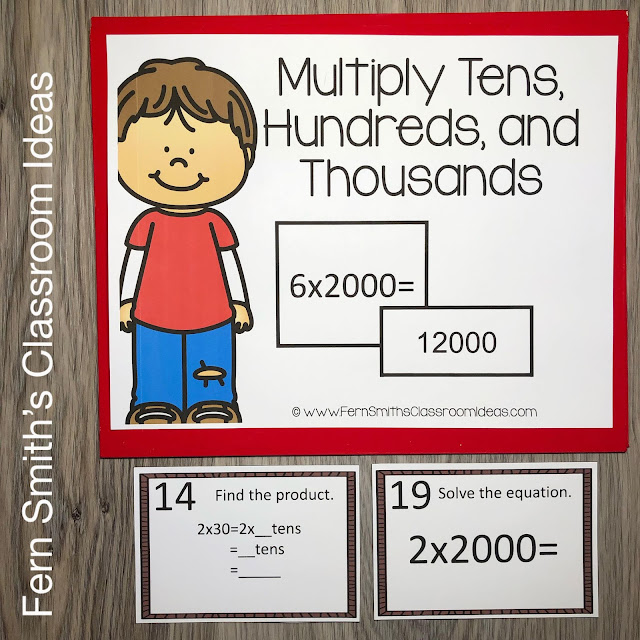My students LOVED having Center Games and Task Cards at our Math Centers and your students will too! You can dedicate two of your math centers (math workstations) as Multiplication Comparisons math centers. By changing out the skill each day, each week, or each month, your students already know the directions for using that math center.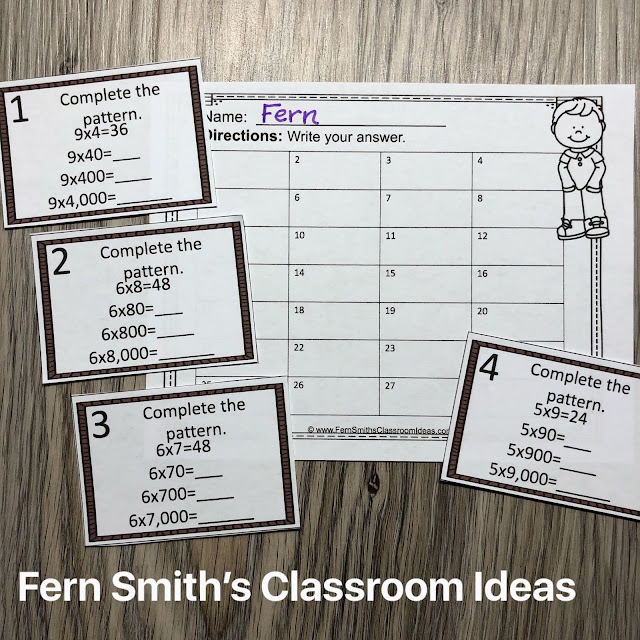Your math task cards and math center games can be placed in a folder with the included folder cover (See my PREVIEW PICTURES) and the students can take the center back to their seats if classroom space is limited. Your students will enjoy the freedom of center games and task cards while learning and reviewing important skills at the same time! This Fourth Grade Math Multiply Tens, Hundreds, and Thousands Center Games and Task Cards Resource Bundle is also perfect for assessment grades for 4th Graders!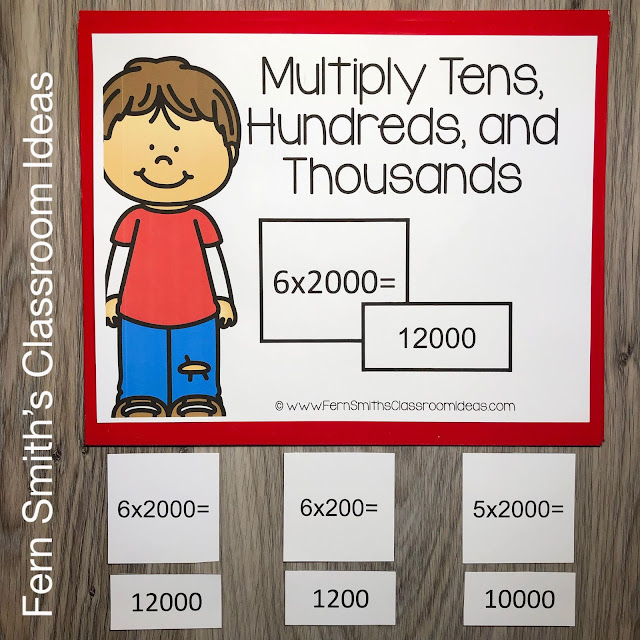Once your students are used to the expectations of using center games and task cards, your math skills math center can run all year with different skills from my TpT Store.

This Fourth Grade Multiply Tens, Hundreds, and Thousands Math Center Games and Task Cards Resource Bundle Contains the Following Resources: has a Matching Task Card Set:

When it comes to the ease of prepping these 4th Grade Multiply Tens, Hundreds, and Thousands Task Cards and Math Center Games, all pieces are rectangular and easy for you or a parent volunteer to cut on a paper slicer. No need to cut around a cute turkey or round pumpkin task card, just rectangles with frames and the math problems, perfect for fourth graders!The Fourth Grade Multiply Tens, Hundreds, and Thousands Math Center Games Resource is Part of the Following Bundles:

This Fourth Grade Multiply Tens, Hundreds, and Thousands Task Cards Resource is Part of the Following Bundles:

This Fourth Grade Multiply Tens, Hundreds, and Thousands Task Cards and Center Games Resource Contains the Following Resources:

The Fourth Grade Multiply Tens, Hundreds, and Thousands Math Task Cards Resource Includes:

Pages 1 - Task Card Folder Cover

Pages 2 to 3 - 8 "Complete the pattern." Multiply Tens, Hundreds, and Thousands Task Cards

Pages 4 to 5 - 8 "Find the product." Multiply Tens, Hundreds, and Thousands Task Cards

Pages 6 to 8 - 8 "Solve the equation." Multiply Tens, Hundreds, and Thousands Task Cards

Page 9 - Recording Sheet for Task Cards 1 to 28.

Page 10 - Answer Key / Student Self-Checking Page.

The Fourth Grade Multiply Tens, Hundreds, and Thousands Math Center Games Resource Includes:

Page One - A colorful cover page stating "Multiply Tens, Hundreds, and Thousands" that can be used for a cover sheet for your file folder in your files. It can also be a math center folder cover for your students, by putting this math center in a folder with the folder cover, your students can take it back to their desk if your classroom space is limited. You can also use the cover page as a poster, placing it on the wall over your math center.

Page Two - A less colorful cover page, saving you colored ink, stating "Multiply Tens, Hundreds, and Thousands" that can be used for a cover sheet for your file folder in your files. It can also be a math center folder cover for your students, by putting this math center in a folder with the folder cover, your students can take it back to their desk if your classroom space is limited. You can also use the cover page as a poster, placing it on the wall over your math center.

Pages Three, Four, & Five - Forty-Eight center game cards with the math problems.

There are many ways to use this 4th Grade Multiply Tens, Hundreds, and Thousands Task Cards and Math Center Games Resource, here are a few of my favorite ways:

• Use one page as a game board, cut the other page. Place all those cards face down. Have the students turn over one card at a time, solve and place face up on the board. If they are incorrect, the card returns to the bottom of the pile. The winner is the student who places the final card. OR, for a "no winner" version, the goal is for the students to solve the cards independently, but to completely cover the board together. This version can ALSO be played two ways. One way is with the answer page as the game board, another way is with the math problem page as the game board instead.
• You can also play this game with cutting all the cards and matching up the problems with the solutions.
• For movement, place the problem cards around the room and the student(s) have to solve the math problem and leave the correct answer card at that location. Have a second student check the answers to extend the game.
• Mix all the cards together, with two students, deal 5 cards to each student and then place the remaining cards face down. Use as a Go Fish card game!
• Lay all the cards upside down on the floor and use as a Concentration (some call it Memory) Game!

Have you ever been given a volunteer one day without advanced notice?

A college student or a local business person maybe that the office assigned to you? These task cards are perfect to give to the volunteer to work one on one with each student during the day. Place a small desk in the corner or in the hallway and your students will love some one on one time practicing their basic facts with the volunteer that day, in addition to making you look like an amazingly prepared teacher! Download this resource today and you'll be ready to go!

*** Excellent for a substitute teacher folder, or sub tub! ***

This 4th Grade Multiply Tens, Hundreds, and Thousands Task Cards and Math Center Games Bundle is also PERFECT for...

• Remediation and RTI groups

• Substitute teacher folders

• Scoot and Read the Room games

• Concentration

• Match Game

• Go Fish

• Assessments

• Fine motor skills work

• Indoor recess

• Morning work

• Emergency sub tubs

• Homework packets

• Math Night with Parents

• Math centers

• Early morning arrival students

• After school tutoring

• Before school tutoring

• And so much more!

This Fourth Grade Multiply Tens, Hundreds, and Thousands Task Cards and Math Center Games Bundle is so easy to prep, it is perfect to give to a parent volunteer who is asking, "How can I help?" or your PTA / PTO volunteer room. It is also terrific for an Emergency Substitute Tub, Folder or Binder!

To Recap 4th Grade Multiply Tens, Hundreds, and Thousands Task Cards and Math Center Games Bundle Includes:

Multiply Tens, Hundreds, and Thousands Task Cards Resource Includes:

Page 1 - Full Color Center Game Folder Cover or Math Center Sign

Pages 2 to 3 - 8 "Complete the pattern." Multiply Tens, Hundreds, and Thousands Task Cards

Pages 4 to 5 - 8 "Find the product." Multiply Tens, Hundreds, and Thousands Task Cards

Pages 6 to 8 - 12 "Solve the equation." Multiply Tens, Hundreds, and Thousands Task Cards

Page 9 - Recording Sheet for Task Cards 1 to 28.

Page 10 - Answer Key / Student Self-Checking Page.

Multiply Tens, Hundreds, and Thousands Center Games Resource Includes:

Page 1 - Full Color Center Game Folder Cover or Math Center Sign

Page 2 - Minimal Color {Save Your Ink} Center Game Folder Cover or Math Center Sign

Pages 3 to 5 - Three Pages of Quick and Easy to Prep Center Game Cards.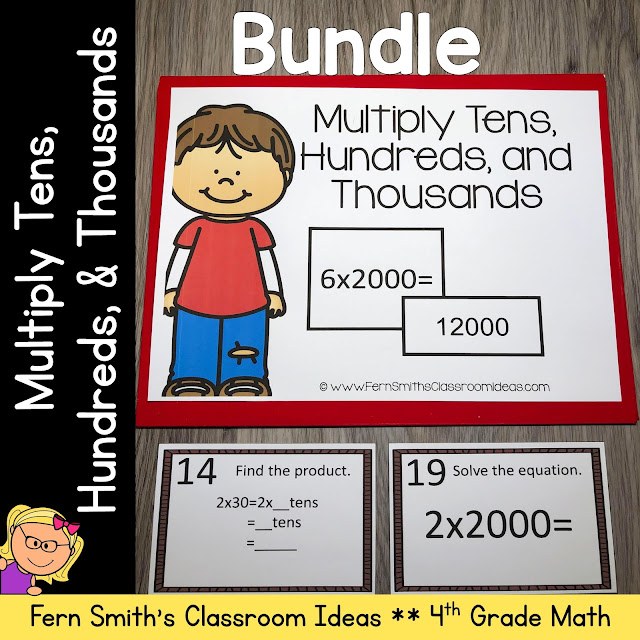Common Core State Standard

CCSS.MATH.CONTENT.4.NBT.A.1

Recognize that in a multi-digit whole number, a digit in one place represents ten times what it represents in the place to its right.

CCSS.MATH.CONTENT.4.NBT.B.5

Multiply a whole number of up to four digits by a one-digit whole number, and multiply two two-digit numbers, using strategies based on place value and the properties of operations. Illustrate and explain the calculation by using equations, rectangular arrays, and/or area models.

Need something great for Fourth Grade?

Click here to see my 4th Grade Math Year Long FULL BUNDLE.

STOP ALL TEXT HERE